I have gSmart mini for some time. This small camera was one of the first very cheep available digital camera knowing 640x480 pixels. How I found through time, Mustek probably pushes it to market too soon, because camera offen hangs and has at all lot of unpredictible bugs. During debugging of gSmart mini driver for Linux, we had main problem with absence of reset. This leads me to break into camera. How you can see, it is not very difficult.

#### Step by step disassemble

1.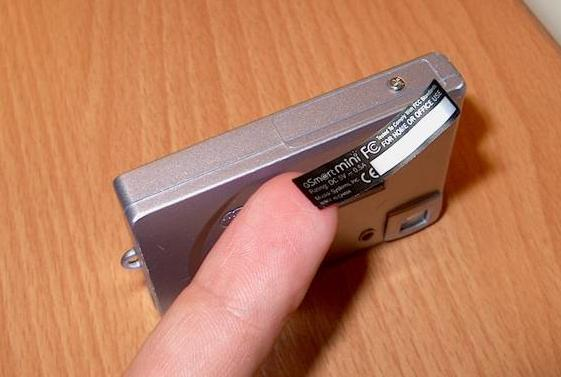2.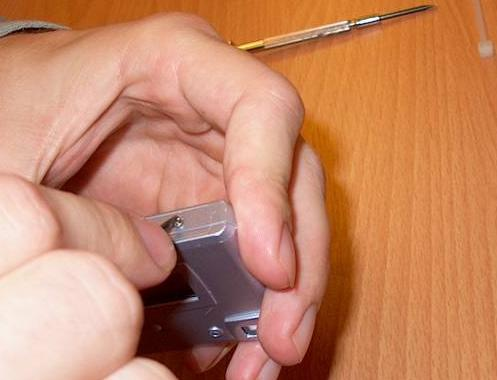3.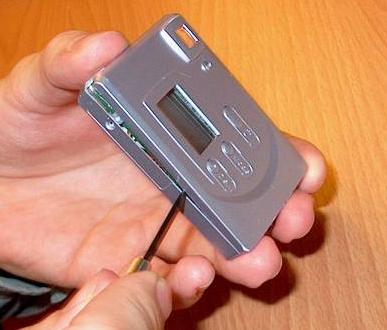4.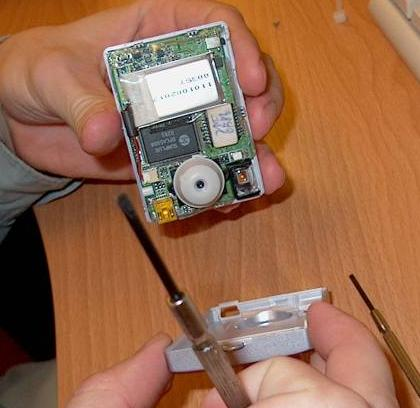5.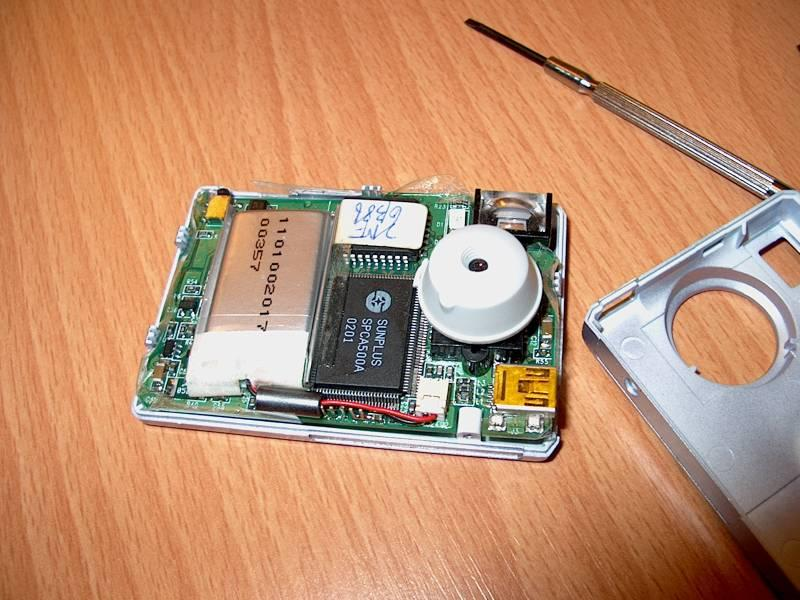6.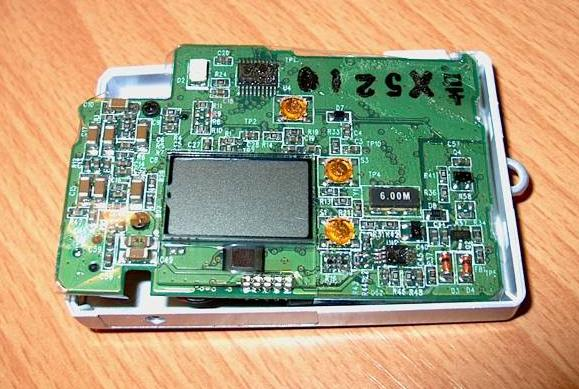#### What important we can get out of it?

• it is not a problem to open it - you need size 0+ and 2.0- screwdrivers
• microprocesor inside is sunplus SPCA500A - hynix demokit with spca500a datasheet
• battery has it's own connector known from other cards too
• reset on spca is unconnected and therefore it is not possible to easily reach it - but we can disconnect battery - we do not need fridge, to freeze camera to reset, anymore! :)

#### My camera USB infos

/proc/bus/usb/device
```T:  Bus=01 Lev=00 Prnt=00 Port=00 Cnt=00 Dev#=  1 Spd=12  MxCh= 2
B:  Alloc=  0/900 us ( 0%), #Int=  0, #Iso=  0
D:  Ver= 1.00 Cls=09(hub  ) Sub=00 Prot=00 MxPS= 8 #Cfgs=  1
P:  Vendor=0000 ProdID=0000 Rev= 0.00
S:  Product=USB UHCI-alt Root Hub
S:  SerialNumber=fcc0
C:* #Ifs= 1 Cfg#= 1 Atr=40 MxPwr=  0mA
I:  If#= 0 Alt= 0 #EPs= 1 Cls=09(hub  ) Sub=00 Prot=00 Driver=hub
E:  Ad=81(I) Atr=03(Int.) MxPS=   8 Ivl=255ms
T:  Bus=01 Lev=01 Prnt=01 Port=00 Cnt=01 Dev#=  4 Spd=12  MxCh= 0
D:  Ver= 1.00 Cls=00(>ifc ) Sub=00 Prot=00 MxPS= 8 #Cfgs=  1
P:  Vendor=055f ProdID=c220 Rev= 0.90
C:* #Ifs= 2 Cfg#= 1 Atr=80 MxPwr=500mA
I:  If#= 0 Alt= 0 #EPs= 1 Cls=ff(vend.) Sub=00 Prot=00 Driver=(none)
E:  Ad=81(I) Atr=01(Isoc) MxPS=   0 Ivl=1ms
I:  If#= 0 Alt= 1 #EPs= 1 Cls=ff(vend.) Sub=00 Prot=00 Driver=(none)
E:  Ad=81(I) Atr=01(Isoc) MxPS= 128 Ivl=1ms
I:  If#= 0 Alt= 2 #EPs= 1 Cls=ff(vend.) Sub=00 Prot=00 Driver=(none)
E:  Ad=81(I) Atr=01(Isoc) MxPS= 384 Ivl=1ms
I:  If#= 0 Alt= 3 #EPs= 1 Cls=ff(vend.) Sub=00 Prot=00 Driver=(none)
E:  Ad=81(I) Atr=01(Isoc) MxPS= 512 Ivl=1ms
I:  If#= 0 Alt= 4 #EPs= 1 Cls=ff(vend.) Sub=00 Prot=00 Driver=(none)
E:  Ad=81(I) Atr=01(Isoc) MxPS= 640 Ivl=1ms
I:  If#= 0 Alt= 5 #EPs= 1 Cls=ff(vend.) Sub=00 Prot=00 Driver=(none)
E:  Ad=81(I) Atr=01(Isoc) MxPS= 768 Ivl=1ms
I:  If#= 0 Alt= 6 #EPs= 1 Cls=ff(vend.) Sub=00 Prot=00 Driver=(none)
E:  Ad=81(I) Atr=01(Isoc) MxPS= 896 Ivl=1ms
I:  If#= 0 Alt= 7 #EPs= 1 Cls=ff(vend.) Sub=00 Prot=00 Driver=(none)
I:  If#= 1 Alt= 0 #EPs= 3 Cls=ff(vend.) Sub=00 Prot=00 Driver=(none)
E:  Ad=82(I) Atr=02(Bulk) MxPS=  64 Ivl=0ms
E:  Ad=03(O) Atr=02(Bulk) MxPS=  64 Ivl=0ms
E:  Ad=84(I) Atr=03(Int.) MxPS=   1 Ivl=8ms

```

#### And now something completely different...

I have probably first gSmart Mini in universe with implemented magnetic reset!
I bought one very small tongue magnetic switch and I add it into ground wire of cameras battery with very small piece of magnet. Now it is necessary to place correctly big magnet near gsmart to disconnect battery.

Also interesting is that camera is not fully reset even if I hold magnet in place for longer time. Only pictures are damaged, but they can be downloaded. Maybe battery is disconected only for a short time because switch is toggled only when magnet is closing.

Umí tohle váš kečup?